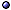Euclidean Geometry

Euclidean Geometry / Space En > s.a. differential geometry / trigonometry.
* Idea: The space $$\mathbb R^n$$ with an affine structure; Choosing an origin and a positive definite quadratic function μ: $$\mathbb R^n \to \mathbb R$$ (which defines an inner product) makes it into a Euclidean vector space.
* Euclidean group: The allowed symmetry transformations (in the sense of Klein's program), the group of rigid motions; In n dimensions, SO(n) $$\times_{\rm s} {\rm T}^n$$.
@ General references: Hilbert 02 [axioms]; Posamentier 02 [advanced].
@ Related topics: Bauer & Wachter EPJC(03)mp/02 [q-deformed]; Soltan 15 [convex sets]; > s.a. decomposition [of tensor fields].
> Online resources: see Euclid's Elements in David Joyce's site; Johan Mebius' page [4D euclidean geometry].

Ellipsoid > s.a. multipole moments.
* Volume: In 3D, V = $$4\over3$$πabc, and for an ellipsoid of revolution (2 equal axes), V = $$4\over3$$π a2b; In n dimensions, multiply the volume of the unit n-sphere by the square root of all the semiaxes.

Polygon > s.a. simplex [including triangle]; Surveyor's Formula.
* Constructible: The ones with 2k, $$3 \cdot 2^k$$, $$5 \cdot 2^k$$, or $$15 \cdot 2^k$$ sides (k ∈ $$\mathbb N$$) are known from BC; The one with 17 sides was found by Gauss.
@ General references Agarwal et al CG(02) [Minkowski sums, algorithms]; James et al JPA(08) [almost convex].
@ Related topics: Charles a0806 [quantization of polygon spaces]; > s.a. markov processes [polygonal Markov fields].
@ In Minkowski space: Foth JGP(08) [3D Minkowski].
* Idea: A subspace of E$$^n$$ made of simplices, whose intersections are faces; A special kind of cell complex.
$Def: The union of all elements of a (locally finite) simplicial complex, together with the Euclidean subspace topology. * Regular polyhedra: The cube, icosahedron, Platonic solids, tetrahedron. * Platonic solids: The five polyhedra in 3D Euclidean space that have equal faces and equal angles at their vertices, the tetrahedron, cube, octahetron, dodecahedron, and icosahedron; There are three generalizations that exist in all dimensions, the hypertetrahedron, hypercube, and hyperoctahedron, with the latter two being dual. * Result (Minkowski): A convex polyhedron is uniquely determined (up to translation) by the directions and areas of its faces. * Examples: Euclidean space $${\mathbb R}^n$$ itself is a polyhedron. @ Platonic solids: Everitt T&A(04) [3-manifolds from identifications]; Dechant ACA-a1307 [4D analogs]; Tavakoli & Gisin a2001 [and tests of quantum mechanics]. @ Related topics: Skarke ht/00-proc, Kreuzer & Skarke RVMP(02)m.AG/00, ATMP(02)ht/00 [reflexive]; Atiyah & Sutcliffe MJM(03)mp [in physics, chemistry and geometry]; Grünbaum DM(07) [polyhedra and graphs]; Montroll 09 [popular level, origami polyhedra]; Koca et al ARP-a1006 [quasi-regular polyhedra and their duals]; Barequet et al CG(13) [polyhedronization of a set of points in $$\mathbb R$$3]; Sellaroli a1712 [reconstructing 3D convex polyhedra from their face normals and areas]; > s.a. Calculating Theorem; Schläfli Formula. @ Variations: Livine JMP(13)-a1307 [framed convex polyhedra as symplectic quotients]; Neiman CQG(13)-a1308 [convex spacelike polyhedra in Minkowski space].Other aspects: see discrete geometry; riemannian geometry / quantum geometry [quantum polyhedra]. Polyhedral Complex > see cell complex; voronoi tiling. Other Concepts and Results > s.a. coordinates; lines; Surfaces. * Curious fact: On E$$^2$$, draw a circle and n points on it in generic positions (vertices of a regular polygon is ok but not necessary); Join all pairs by a line; This divides the disk into N(n) regions; For n = 1, 2, 3, 4, 5, N(n) = 1, 2, 4, 8, 16; What is the next one? Answer: 31. [N(n) is given by some known polynomial.] @ References: Topper & Vincent pt(17)dec [Einstein's solution of a high-school geometry problem]. > Related concepts and results: see Cassini Oval; conical sections (ellipse, hyperbola, parabola); Pythagorean Theorem; simplex; spheres; Spiral. Euclidean Metric on a Manifold > a.k.a. riemannian geometry.$ Def: Given a vector bundle (E, π, M), a map μ: E → $$\mathbb R$$ making each fiber into a Euclidean vector space.